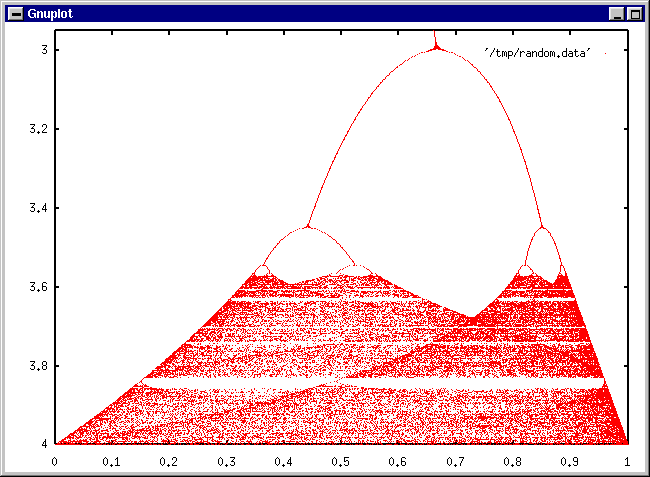#Chaos Theory and Iterative Systems

Math Lair Home > Topics > Chaos Theory and Iterative Systems

"For want of a nail, the shoe was lost.
For want of a shoe, the horse was lost.
For want of a horse, the rider was lost.
For want of a rider, the battle was lost.
For want of a battle, the kingdom was lost."

An iterative system is simply a function where the output for the next step, or iteration, is dependent on the the result from the previous iteration. This sort of system can be applied to real life, where all sorts of things depend on their current state. For example, the number of human beings alive in the year 2011 depends in some way on the number of human beings alive in the year 2010. The more people that are alive this year, the more babies will be born by next year. This effect works both ways, though. The more people are alive, the less space there is on Earth for people, so once the human population gets beyond a certain point (which it certainly hasn't reached yet, since the population still seems to be growing exponentially), the growth rate will be negatively affected by the population.

A simpler example would be the number of people infected by a contagious disease over time. The more people who have the disease, the more people there are to spread it, but there are fewer people to catch it, since there are more immune people now.

These phenomena can be modelled fairly well by the following mathematical model:
xn+1 = rxn(1 - xn)
This is the famous logistic equation that was introduced in 1845 by the Belgian mathematician/sociologist Pierre-Francois Verhulst to model the growth of populations that are limited by finite amounts of resources (food, area, etc.). Here n is an integer starting at zero, and r is a factor between 0 and 4 represents the rate of increase. A higher level of r means that the population increases with greater rapidity.

What happens to the population under this model? Here's a graph (which I made using the program gnuplot) which shows what happens:This requires a bit of explanation. At the top of the graph are iterations using lower values of r. These iterations tend to eventually settle around one value, as the one point for each r value shows. If r is increased enough, the final population level does not settle, but oscillates between two values (this is the area where the curve at the top breaks into two). If r gets even higher, then the population will oscillate between four values, eight values, but if r increases even further (to be precise, if the value of r exceeds 3.56994571869), all order appears to disappear, and the population loops between many different values. These values correspond to those vast red areas at the bottom of the graph. These are the chaos regions of this function.

Chaos is sometimes referred to as the "butterfly effect"; weather patterns are an example of iterative systems that can exhibit chaotic behaviour, so, conceivably, if a butterfly were to flap its wings in Japan, that might make a difference to result in a hurricane in the North Atlantic months later.

Fractals are related to iterative systems.